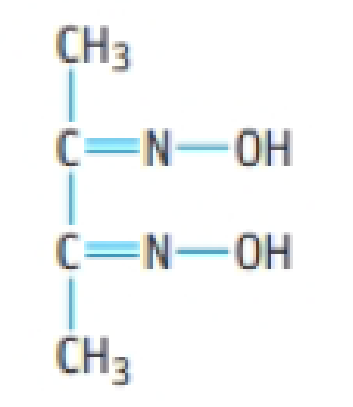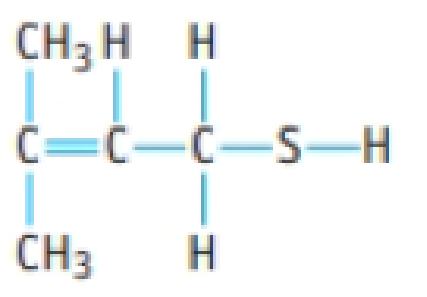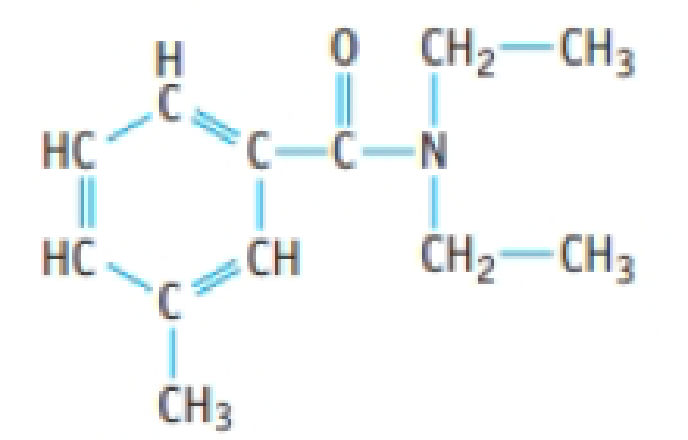Chapter 2, Problem 74PS

Chapter
Section
Textbook Problem

Assume you have 0.123 mol of each of the following compounds. What mass of each is present?(a) C14H10O4, benzoyl peroxide, used in acne medications(b) Dimethylglyoxime, used in the laboratory to test for nickel(II) ions(c) The compound below, responsible for the "skunky" taste in poorly made beer.(d) DEFT, a mosquito repellent(a)

Interpretation Introduction

Interpretation:

For the given amounts of molecules in moles, the mass conversions are needed to be calculated.

Concept introduction:

Molar mass of benzoyl peroxide, dimethylglyoxime, unnamed compound and DEET are 242.23, 116.12, 104.21 and 191.27.

• Conversion formula for mass of an atom into number moles,

Numberofmoles×Molarmass=Massingrams

Explanation

The amount of benzoyl peroxide is given as 0.123mol.

Molar mass of benzoyl peroxide is 242.23g/mol.

Conversion formula for number moles of an atom into mass in grams,

Numberofmoles×Molarma

(b)

Interpretation Introduction

Interpretation:

For the given amounts of molecules in moles, the mass conversions are needed to be calculated.

Concept introduction:

Molar mass of benzoyl peroxide, dimethylglyoxime, unnamed compound and DEET are 242.23, 116.12, 104.21 and 191.27.

• Conversion formula for mass of an atom into number moles,

Numberofmoles×Molarmass=Massingrams

(c)

Interpretation Introduction

Interpretation:

For the given amounts of molecules in moles, the mass conversions are needed to be calculated.

Concept introduction:

Molar mass of benzoyl peroxide, dimethylglyoxime, unnamed compound and DEET are 242.23, 116.12, 104.21 and 191.27.

• Conversion formula for mass of an atom into number moles,

Numberofmoles×Molarmass=Massingrams

(d)

Interpretation Introduction

Interpretation:

For the given amounts of molecules in moles, the mass conversions are needed to be calculated.

Concept introduction:

Molar mass of benzoyl peroxide, dimethylglyoxime, unnamed compound and DEET are 242.23, 116.12, 104.21 and 191.27.

• Conversion formula for mass of an atom into number moles,

Numberofmoles×Molarmass=Massingrams

Still sussing out bartleby?

Check out a sample textbook solution.

See a sample solution

The Solution to Your Study Problems

Bartleby provides explanations to thousands of textbook problems written by our experts, many with advanced degrees!

Get Started